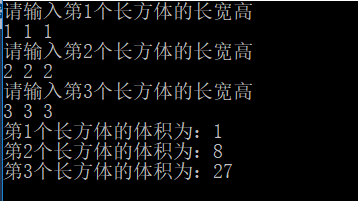需要求3个长方体的体积，请编写一个基于对象的程序。C++语言版本

（1）由键盘分别输入3个长方体的长宽高；

（2）计算长方体的体积；

（3）输出3个长方体的体积；

/*

（1）由键盘分别输入3个长方体的长宽高；
（2）计算长方体的体积；
（3）输出3个长方体的体积；
*/
#include <iostream>
using namespace std;

//Rctangle    ：长方形
class Rectangle {
private:
double length;
double width;
double heigth;
public:
double showVolume(){
double Volume = length * width * heigth;//长方体体积=长*宽*高
return Volume;
}
void setValue() {
cin >> length >> width >> heigth;
}
};

int main() {
Rectangle R;
int i;
for (i = 0; i < 3; i++) {
cout << "请输入第" << i+1 << "个长方体的长宽高" << endl;;
R[i].setValue();
}
for (i = 0; i < 3; i++) {
cout <<"第"<<i+1<< "个长方体的体积为：";
cout << R[i].showVolume() << endl;;
}
return 0;
}//Rctangle    ：长方形
class Rectangle {
private:
double length;
double width;
double heigth;
public:
double showVolume(){
double Volume = length * width * heigth;//长方体体积=长*宽*高
return Volume;
}
void setValue() {
cin >> length >> width >> heigth;
}
};

int main() {
Rectangle R;
int i;
for (i = 0; i < 3; i++) {
cout << "请输入第" << i+1 << "个长方体的长宽高" << endl;;
R[i].setValue();
}
for (i = 0; i < 3; i++) {
cout <<"第"<<i+1<< "个长方体的体积为：";
cout << R[i].showVolume() << endl;;
}
return 0;
}